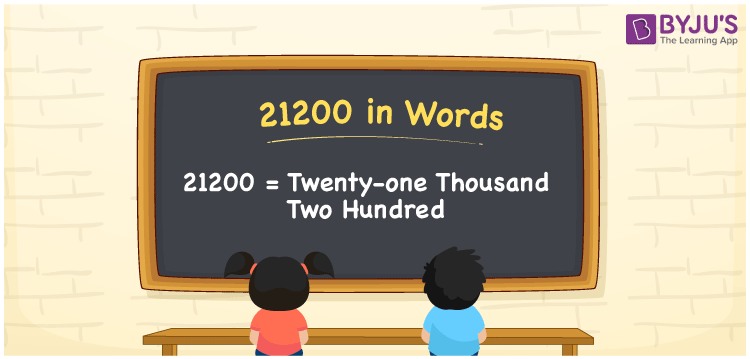# 21200 in Words

We can write 21200 in words as Twenty-one thousand Two hundred. We can easily convert the numeral 21200 into words with the help of a place value chart. Also, we know that the word form of a number is simply the number name. That means Twenty-one thousand Two hundred is the number name for 21200. Thus, number names are used to spell or read the given number.

 21200 in words Twenty-one thousand Two hundred Twenty-one thousand Two hundred in Numbers 21200

## 21200 in English words

Generally, we can write numbers in words using the English alphabet. Thus, we can read 21200 in English as “Twenty-one thousand Two hundred”.## How to Write 21200 in Words?

To derive the word form of the number 21200, we must create a place value chart with five columns as it has five digits. This can be done as follows.

 Ten thousands Thousands Hundreds Tens Ones 2 1 2 0 0

Here, ones = 0, tens = 0, hundreds = 2, thousands = 1, ten thousands = 2

By expanding these digits according to their place values, we get;

2 × Ten thousand + 1 × Thousand + 2 × Hundred + 0 × Ten + 0 × One

= 2 × 10000 + 1 × 1000 + 2 × 100 + 0 × 10 + 0 × 1

= 20000 + 1000 + 200

= Twenty thousand + One thousand + Two hundred

= Twenty-one thousand Two hundred

Hence, 21200 in words = Twenty-one thousand Two hundred.

As we know, 21200 is a natural number that is the successor of 21199 and the predecessor of 21201.

21200 in words – Twenty-one thousand Two hundred

Is 21200 an odd number? – No

Is 21200 an even number? – Yes

Is 21200 a perfect square number? – No

Is 21200 a perfect cube number? – No

Is 21200 a prime number? – No

Is 21200 a composite number? – Yes

## Frequently Asked Questions on 21200 in Words

Q1

### How do you write 21200 in words?

We generally write the number 21200 in English words as Twenty-one thousand Two hundred.
Q2

### What is the value of Twenty-one thousand Two hundred minus Ten thousand two hundred?

Twenty-one thousand Two hundred minus Ten thousand two hundred = 21200 – 10200 = 11000.
Q3

### How do you convert 21200 in words?

We can convert the 21200 into words by writing its expanded form, as given below: 21200 = 20000 + 1000 + 200 = 21000 + 200 = Twenty-one thousand + Two hundred = Twenty-one thousand Two hundred.